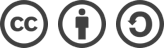## 算法两数之和c#版本

### 算法两数之和c#版本

``````输入：(2 -> 4 -> 3) + (5 -> 6 -> 4)

#### 1. 创建一个链表用于计算

``````public class LinkNode
{
public int val;
{
val = x;
}

}``````

#### 算法实现

1.声明一个链表初始化为0，最后返加.next就可以。

``````public class Solution
{
{
LinkNode p = l1, q = l2, currNode = result;
int carry = 0;
while (p != null || q != null)
{
int x = (p != null) ? p.val : 0;
int y = (q != null) ? q.val : 0;
int sum = x + y + carry;
carry = sum/10;
currNode = currNode.next;
if (p != null ) p = p.next;
if (q != null ) q = q.next;
}
if (carry > 0)
{
}
return result.next;
}
}``````

#### 测试：

``````static void Main(string[] args)
{
Console.WriteLine(result.val);
Console.WriteLine(result.next.val);
Console.WriteLine(result.next.next.val);
Console.WriteLine(result.next.next.next.val); //当向最高位进位时，调用